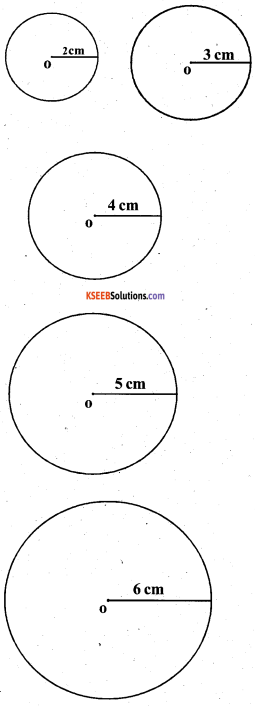# KSEEB Solutions for Class 5 Maths Chapter 7 Circles

Students can Download Maths Chapter 7 Circles Questions and Answers, Summary, Notes Pdf, KSEEB Solutions for Class 5 Maths helps you to revise the complete Karnataka State Board Syllabus and score more marks in your examinations.

## Karnataka State Syllabus Class 5 Maths Chapter 7 Circles

### KSEEB Class 5 Maths Circles Ex 7.1

I. Complete the following using suitable
a) The distance between the centre of a circle and a point on the circle is called radius.
b) In the given figure,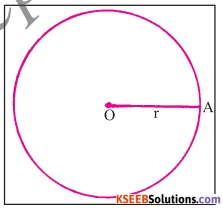1. Centre of the circle is O
2. Radius of the circle is represented by the line segment OA
3. Radius of the circle is rII. Mark the correct statement by (✓) and false statement by (✘)

a) Only one radius can be drawn to a circle (✘)
b) All radii of a circle are equal (✓)
c) There is only one centre for a circle (✓)
d) In the figure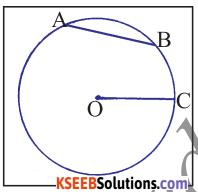i OC is the measure of the radius (✓)
ii. AB is the radius (✘)III. i) in each of the following figures, identify the circle, its radius and centre.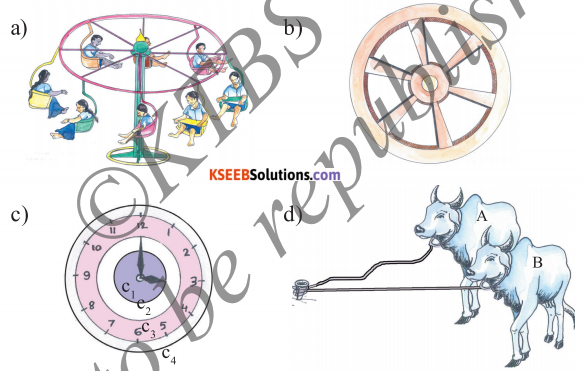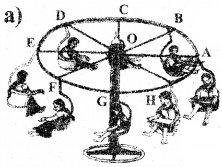O – is the centre
Radii – OA, OB, OC, OD, OE, OF, OG, OH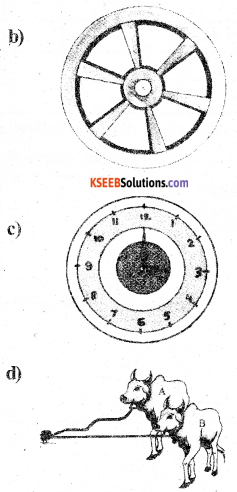ii) In figure (c), how many circles are there? Do they have the same centre?

Four, yes

iii) In figure (c), the length of the needle which is showing the hour is the radius ______ of circle.
(c) No (d) Yes (e) OB

iv) In figure (d), the length of the needle which is showing the minute is the radius of ______ circle.

v) in figure (d), which cow’s thread will represent the radius of the circle?
___________. OBIV. Measure the length of the radius of each of the following circles. Write them in the given space: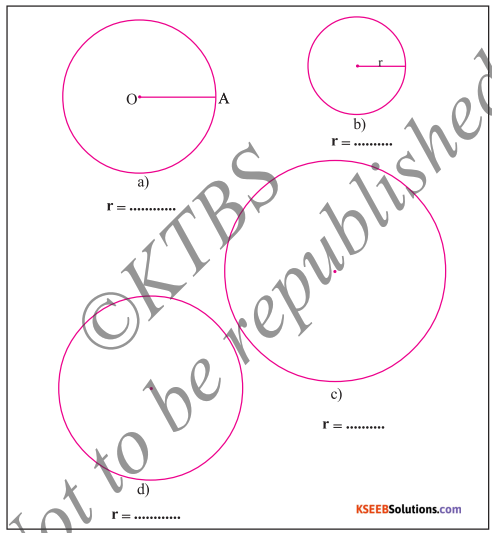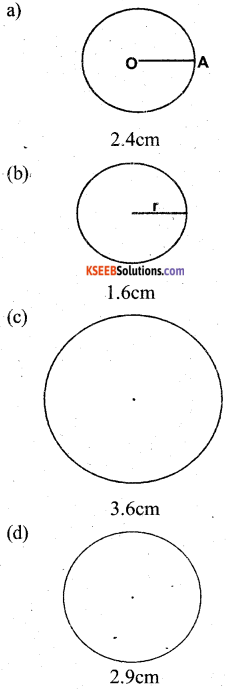### KSEEB Class 5 Maths Circles Ex 7.2

1. Construct the circles with the following radii.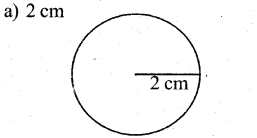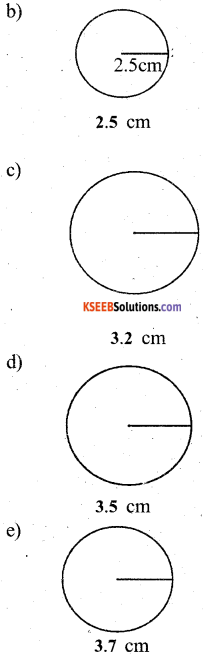2. The students in the following figures have to draw circles with the given radii. So the students have placed the composes on the scale as shown. If they have placed the compasses correctly put ‘✓’ if not put ‘✘’. Take correct measurements and construct the circles in your book.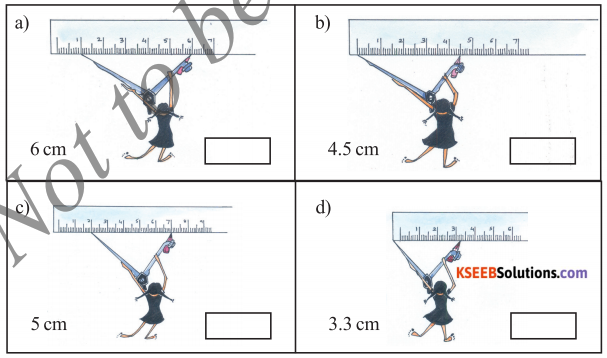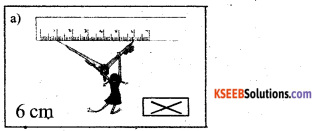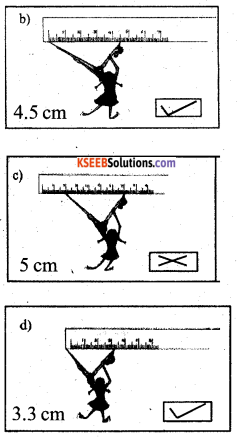3. Observe the figures given below. Write the name of geometrical instruments required to construct them, against the space provided.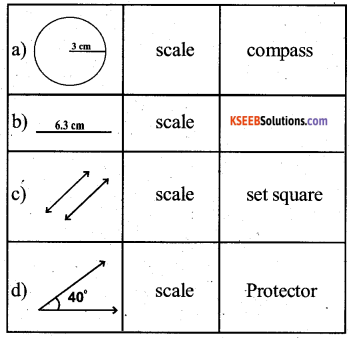4. ‘O’ Mark a point O. With O as centre draw circles with radii 2cm, 3cm, 4cm, 5cm and 6cm.